﻿ Introduction to Variables
INTRODUCTION TO VARIABLES
• PRACTICE (online exercises and printable worksheets)
Want more details, more exercises? Read the full text!

Mathematicians can't do their job without variables!
Variables are used to hold objects; we get to specify the objects to be held.

Here's the precise definition:

DEFINITION variable, universal set
A variable is a symbol (usually a letter) that is used to represent a member of a specified set.
This “specified set” is called the variable's universal set.The universal set for a variable is its supplier—its universe:
the variable is allowed to hold anything that lives in its universal set.
In other words, what the variable holds is allowed to vary over the entire universal set;
hence the name variable is appropriate.

THREE COMMON USES FOR VARIABLES

• to state a general principle
Example:   For all real numbers $\,x\,$ and $\,y\,$, $\,x+y = y + x\;$.
• to represent a sequence of operations
Example:   The expression $\,2x+3\,$ represents the sequence of operations “take a number, multiply by $\,2\,$, then add $\,3\,$”.
• to represent something that is currently unknown, but that we would like to know
Example:   Solve for $\,x\,$:   $\,2x+3 = 5\,$
To “solve for $\,x\,$” means to find the value of $\,x\,$ that makes the sentence true.
Here, the solution is $\,x = 1\,$ since $\,2(1) + 3 = 5\,$.

SHORTHAND FOR MULTIPLICATION: JUXTAPOSITION

Juxtaposition refers to positioning things side-by-side.
When using variables, multiplication can be denoted by juxtaposition.
The expression $\,xy\,$ means $\,x\,$ times $\,y\,$.
The expression $\,2x\,$ means $\,2\,$ times $\,x\,$.
Thus, when no confusion can result, the centered dot that denotes multiplication is usually dropped.
Of course, $\,2\cdot 3\,$ cannot be shortened to $\,23\,$, since this would be confused with the base ten number twenty-three!

Furthermore, whenever a variable (like $\,x\,$) is being multiplied by a specific number (like $\,2\,$),
then it is conventional to write the specific number first.
That is, write $\,2x\,$, not $\,x2\,$.
As you read mathematics, start noticing that whenever a specific number is multiplying a variable,
the specific number is always written first.

CONVENTIONS IN MATHEMATICS

The English language has lots of conventions.
For example, the capitalization of proper nouns clues the reader that Carol refers to a person,
whereas carol refers to a Christmas song.

Mathematics has lots of conventions regarding the naming of variables,
which help clue the reader to the type of objects the variable can hold.

Numbers are usually represented by lowercase letters.
Sets are usually represented by uppercase letters.
(Memory device: sets are collections of things—they're big—so we use big letters to represent them.)

Notice that the symbol $\,\mathbb{R}\,$ (blackboard bold R, used to represent the set of real numbers)
and the symbol $\,\mathbb{Z}\,$ (blackboard bold Z, used to represent the set of integers)
are both uppercase letters (in a special typestyle).

A variable with universal set $\,\mathbb{R}\,$ (or, any interval of real numbers)
is most likely to be named with a lowercase letter from the end of the alphabet,
particularly $\,t\,$, $\,x\,$, or $\,y\,$.

A variable with universal set $\,\mathbb{Z}\,$ (or, any subset of the integers)
is most likely to be named with a lowercase letter from the middle of the alphabet,
particularly $\,i\,$, $\,j\,$, $\,k\,$, $\,m\,$, or $\,n\,$.
MEMORY DEVICE:   The I Ntegers use letters from $\,i\,$ to $\,n\,$.

These conventions are summarized in the diagram below: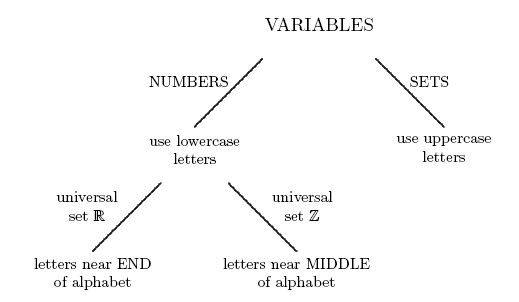Remember that the ultimate choice of letter used for a variable is up to you.
You can override any convention simply by informing people of your intentions.
Keep in mind, however, that an awareness of mathematical conventions provides valuable clues in reading mathematics.
If you (mostly) adhere to normal conventions in your own mathematical work, then you're apt to have a happier audience.

HAND-WRITING VARIABLES

Whenever letters are used in a mathematical context (i.e., as variables), they are typeset in an italic style.
This convention helps to visually distinguish letters being used in a mathematical way from letters being used in a non-mathematical way.

When hand-writing mathematics, it's particularly easy to confuse variables with other things, as the following cautions indicate:
Don't write $\,x\,$ as; it can be confused with a multiplication symbol.
Don't write $\,y\,$ as; it can look like an ex ($\,x\,$).
Don't write $\,z\,$ as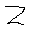; it can look like the number $\,2\,$.
Don't write $\,t\,$ as; it can look like a plus sign.
Don't write $\,i\,$ as; dots get lost, and then it looks like the number one.
Don't write $\,l\,$ as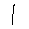; it can look like the number one.

For these reasons, when hand-writing mathematics, you'll want to try and duplicate an italic font, as illustrated in the following table:

typestyle used in English words typestyle used for variable how to hand-write
x $\,x\,$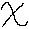y $\,y\,$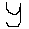z $\,z\,$t $\,t\,$i $\,i\,$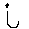j $\,j\,$k $\,k\,$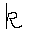l $\,l\,$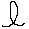m $\,m\,$n $\,n\,$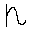Master the ideas from this section